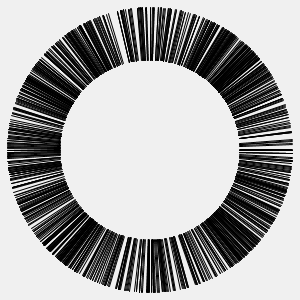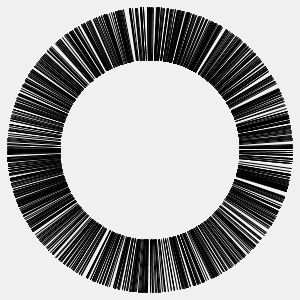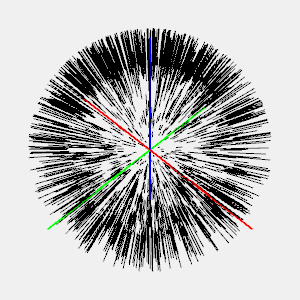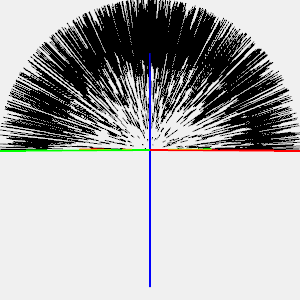# Random Vector Generation

In this article, I want to discuss unit vector generation. It was inspired by the recent addition of random-2d and random-3d functions in Quil API. The goal is simple: write a function that generates a random unit vector, both in 2D and 3D. This article doesn’t use advanced math (e.g. statistics) and discusses the matter from software engineer point of view who forgot college course about statistics and theory around generating random values.

### 2D vectors

Let's start with the 2D. Here the naive approach that I would try first:

1. Generate `x` and `y` as random numbers from `[-1, 1]`.
2. Normalize vector: divide `x` and `y` by the vector length to make it a unit vector.

Clojure implementation:

``````(defn rand-2d []
(let [x (dec (rand 2))
y (dec (rand 2))
len (Math/sqrt (+ (* x x) (* y y)))]
[(/ x len) (/ y len)]))
``````

Now let’s see how well it works in practice. To do that let’s generate 2000 vectors and draw them. When drawing I "cut" beginning of vectors to make image cleaner:It's not obvious, but you can see that there are more vectors in the "corners" at 45°, 135°, 225° and 315° and fewer vectors at 0°, 90°, 180° and 270°. You can start animation to see better. This clustering doesn’t seem good. Good vector generator must generate uniformly distributed vectors.

Let's try another approach that uses the fact that unit vector is "almost" defined by just one of its coordinate, x or y. Given x we can calculate y as ±√(1 - x²). So let’s try this approach: 1. Generate `x` as random number from [-1, 1]. 2. Calculate `y` as `sqrt(1 - x * x)`. 3. Multiply `y` by -1 with 0.5 chance otherwise, y will be always positive.

Clojure implementation:

``````(defn rand-2d []
(let [x (dec (rand 2))
sign (rand-nth [-1 1])
y (* sign (Math/sqrt (- 1 (* x x))))]
[x y]))
``````

Visualization:Turns out it's even worse than the first approach. At least now we can clearly see that it's bad.

Let's try yet another approach. In the first and second approaches we saw vectors clustered around certain angles. So we need to make angles uniformly distributed. To achieve that instead of generating x and y we’ll randomly choose an angle and calculate x and y based on the angle. In other words we'll generate a unit vector in polar coordinates instead of cartesian. In cartesian coordinates vector is defined by a pair [x, y] while in polar the pair is [r, ϕ] where r is the vector length and ϕ is the angle:Third (polar) approach:

1. Set `r = 1` (unit vector). Generate ϕ as random number from `[0, 2π]`
2. Calculate `x = r*cos(ϕ)`, `y = r*sin(ϕ)`

Clojure implementation:

``````(defn rand-2d []
(let [phi (rand (* 2 Math/PI))]
[(Math/cos phi)
(Math/sin phi)]))
``````

Visualization:Finally it looks good. Comparing with the first approach vectors feel more uniformly distributed.

### 3D vectors

Great, we figured out 2D. What about 3D then? The situation is very similar. Let’s try the first (naive) approach;

1. Generate `x`, `y`, `z` as random numbers from `[-1, 1]`.
2. Normalize vector: divide `x`, `y` and `z` by the vector length to make it unit vector.

Clojure implementation:

``````(defn rand-3d []
(let [x (dec (rand 2))
y (dec (rand 2))
z (dec (rand 2))
len (Math/sqrt (+ (* x x) (* y y) (* z z)))]
[(/ x len) (/ y len) (/ z len)]))
``````

Visulization:Unfortunately my visualization sucks. I couldn't come up with a better technique to draw vectors in 3D. Red/green/blue lines are X/Y/Z axis. It's kinda hard to see if the vectors uniformly distributed or not. Let's try other approaches. I'll skip code for the second approach (it's equivalent to 2D), here is the visualization:As for the third approach, in 3D we have to use spherical coordinates. In spherical coordinates vector is defined by three numbers (r, φ, θ):The algorithm is the following:

1. Set `r = 1`. Generate `θ` as random number from `[-π/2, π/2]`, φ from `[0, 2π]`.
2. Calculate `x = r*cos(θ)*cos(φ)`, `y = r*cos(θ)*sin(φ)`, `z = r*sin(θ)`.

Clojure implementation:

``````(defn rand-3d-v3 []
(let [phi (rand (* 2 Math/PI))
theta (- (rand Math/PI) (/ Math/PI 2))]
[(* (Math/cos theta) (Math/cos phi))
(* (Math/cos theta) (Math/sin phi))
(Math/sin theta)]))
``````

Visualization:Results surprised me. My mind tells me that the third approach is the best, but my eyes prefer the first approach. The visualization from the first approach feels more uniform, I see fewer clusters. But maybe it's inefficiencies of my 3D visualization: some vectors are close to the camera and they look bigger and more clustered while the ones which further look smaller. If you have ideas how to fix it - please leave comments. I'm curious how better I can visualize it.

### Conclusion

If you need to generate random vectors think carefully if your approach produces uniformly distributed vectors (if it matters for your application). I like the approach with polar/spherical coordinates because I can "see" it. If you google around you'll find suggestions to use gaussian distribution but to me it's harder to understand.

Also while working on article I found embarrassing things:

1. `random-2d` and `random-3d` were supposed to be released in Quil 2.6.0 but they're not there. Apparently I built the release from earlier commit.
2. Quil `random-3d` has bug and it produces only vectors with positive z coordinate:If you want to play with generation and animation you can use Quil sketch editor for Clojurescript: 2D sketch and 3D sketch. If you prefer Clojure - checkout this project.

Published on 09 Apr 2017